Author: Oscar Cronquist Article last updated on May 05, 2022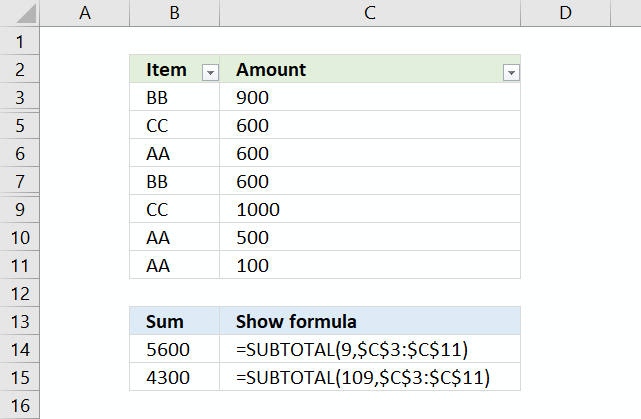The picture above shows row 4 and row 8 hidden, the SUM argument 9 sums all values in C3:C11 whereas argument 109 ignores hidden values. That is why you see different sums in cell B14 and B15.

## 1. SUBTOTAL function syntax

SUBTOTAL(function_num, ref1, ...)

The function returns a subtotal from a list or database, you can choose from a variety of arguments that determine what you want the function to do.

The SUBTOTAL function allows you to have up to 254 cell ranges.

## 2. SUBTOTAL function arguments

Filtered-out cells from Excel Tables and Excel feature "Filter" are always excluded.

 Function_num (includes hidden values) Function_num (ignores hidden values) Function 1 101 AVERAGE 2 102 COUNT 3 103 COUNTA 4 104 MAX 5 105 MIN 6 106 PRODUCT 7 107 STDEV 8 108 STDEVP 9 109 SUM 10 110 VAR 11 111 VARP

To clarify, hidden values are manually hidden values. Filtered-out values are not manually hidden values.

## 3. Does the SUBTOTAL function avoid double counting?

Yes, other SUBTOTAL formulas in your cell range are ignored to avoid double counting.

## 4. How to include manually hidden values in the SUBTOTAL function?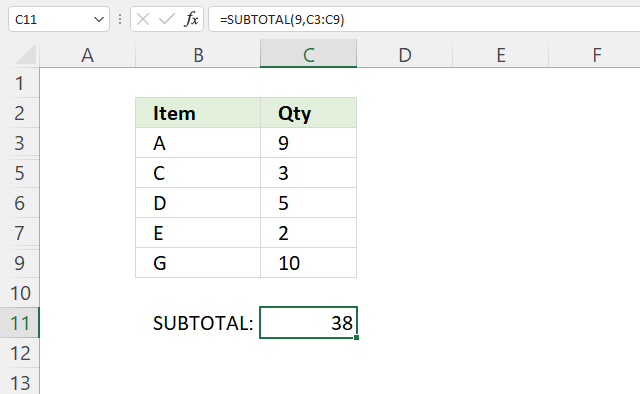Use function_num between 1 and 11 to include manually hidden values to the SUBTOTAL function.

Formula in cell C11:

=SUBTOTAL(9, C3:C9)

Rows 4 and 8 are hidden. The total is 38: 9 + 7 + 3 + 5 + 2 + 2 + 10 = 38.

Cell C4 contains 7 and cell C8 contains 2. The first argument is 9 which is between 1 and 11 meaning manually hidden cells are included.

## 5. How to exclude manually hidden values from the SUBTOTAL function?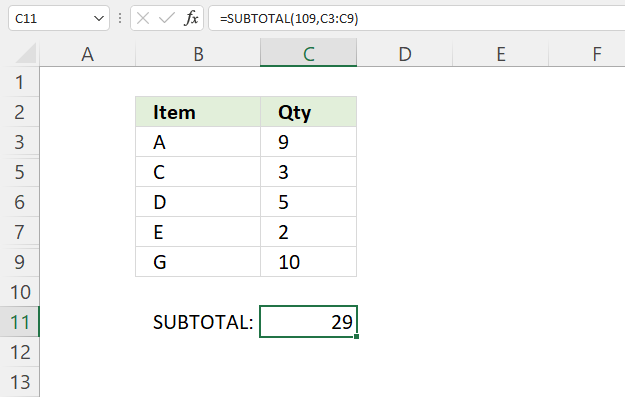Use function_num between 101 and 111 to exclude manually hidden values to the SUBTOTAL function.

Formula in cell C11:

=SUBTOTAL(109, C3:C9)

The formula in cell C11 excludes manually hidden values in the SUBTOTAL calculation. Rows 2 and 8 are manually hidden.

9 + 3 + 5 + 2 + 10 equals 29.

## 6. How to sum filtered data?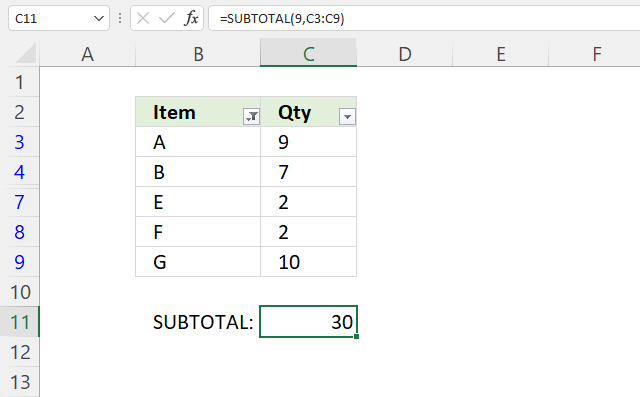The image above demonstrates a formula that adds number and returns a total based on filtered values.

I have applied "Filter" to cell range B2:C9, the buttons next to the column header names show that you can apply a filter. The data table has rows 5 and 6 filtered out.

Formula in cell C11:

=SUBTOTAL(9, C3:C9)

The first argument is 9 which represents the SUM function, it adds numbers and returns a total.

9 + 7 + 2 + 2 + 10 equals 30 which is all visible numbers in cell range C3:C9.

## 7. How to count the number of cells containing numbers based on filtered data?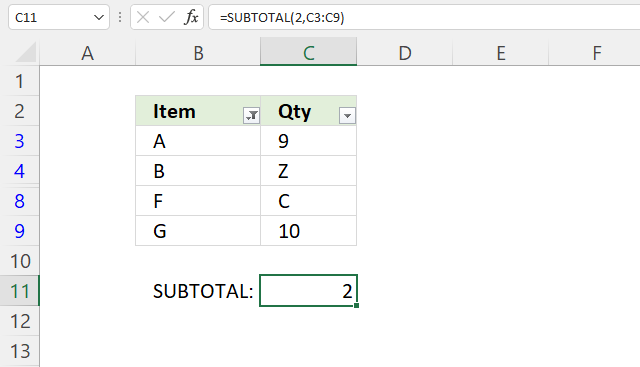The image above demonstrates a formula in cell C11 that counts the number of numbers based on filtered values in a given cell range.

Cell range C3:C9 contains both numbers and letters and is filtered. Cells C5, C6, and C7 are filtered out.

Formula in cell C11:

=SUBTOTAL(2, C3:C9)

The COUNT function counts the number of numbers in a given cell range. There are two visible numbers in cell range C3:C9.

## 8. How to count the number of not empty cells based on filtered data?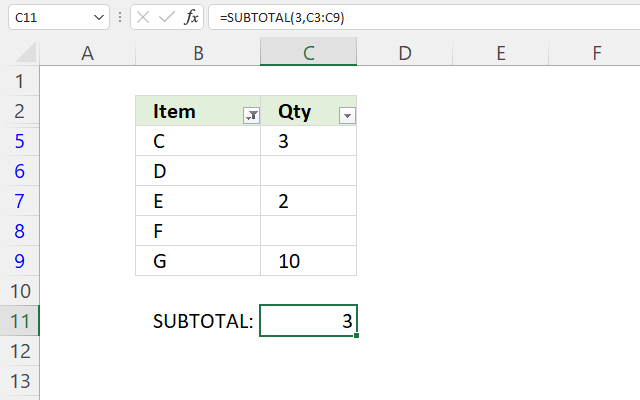The image above shows a filtered data set in cell range B3:C9, it has a few empty cells. The formula in cell C11 counts not empty cells based on the filtered data.

Formula in cell C11:

=SUBTOTAL(3, C3:C9)

The first argument is 3 which represents the COUNTA function, it counts not empty cells.

Rows 3 and 4 are filtered out. Cells C5, C7, and C9 are not empty. The formula returns 3.

## 9. How to extract the largest number from filtered data?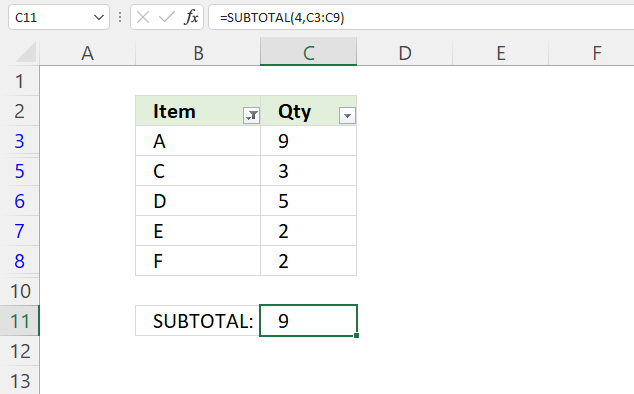The picture  above shows filtered data in cell range B3:C9, the formula in cell C11 extracts the largest number from the filtered cells.

Formula in cell C11:

=SUBTOTAL(3, C3:C9)

The first argument is 4 which represents the MAX function, it extracts the largest number.

## 10. How to extract the smallest number from filtered data?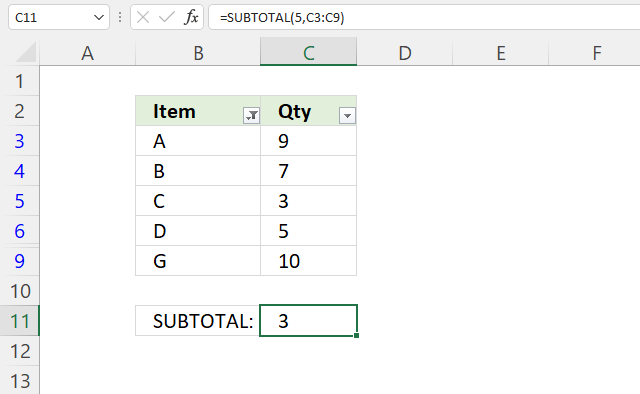The image above shows filtered data in cell range B3:C9, the formula in cell C11 extracts the smallest number from the visible cells.

Formula in cell C11:

=SUBTOTAL(4, C3:C9)

The first argument is 5 which represents the MIN function, it extracts the largest number.

## 11. How to calculate an average based on filtered data?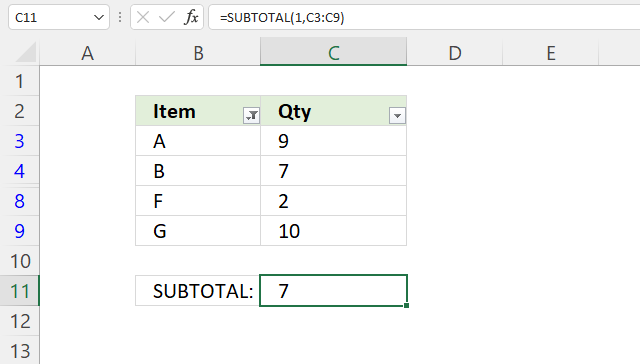The image above shows filtered data in cell range B3:C9, the formula in cell C11 calculates the average number based on the visible cells in cell range C3:C9.

Formula in cell C11:

=SUBTOTAL(1, C3:C9)

The first argument is 1 which represents the AVERAGE function, it calculates the average based on a group of numbers.

9 + 7 + 2 + 10 = 28. 28/4 = 7.

## 12. Extract filtered values - Excel 365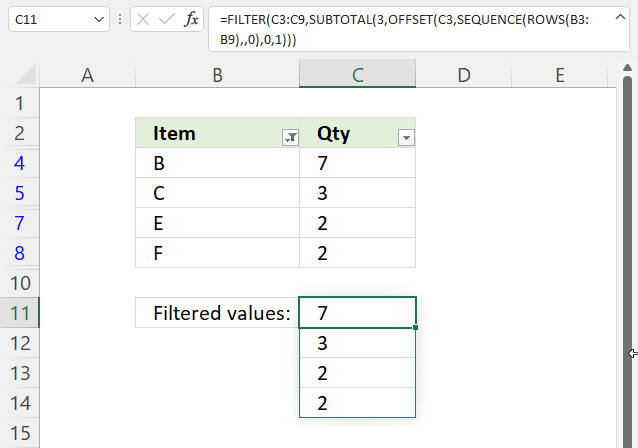The image above shows an Excel 365 formula in cell C11 that extracts filtered values from cell range C3:C9.

Formula in cell C11:

=FILTER(C3:C9, SUBTOTAL(3, OFFSET(C3, SEQUENCE(ROWS(B3:B9), , 0), 0, 1)))

### Explaining formula in cell C11

#### Step 1 - Calculate rows in cell range

The ROWS function calculates the number of rows a cell range contains.

ROWS(B3:B9)

returns 7. Cell range B3:B9 contains seven rows.

#### Step 2 - Create a sequence from 0 (zero) to n

The SEQUENCE function creates a list of sequential numbers to a cell range or array. It is only available to Excel 365 subscribers.

SEQUENCE(rows, [columns], [start], [step])

SEQUENCE(ROWS(B3:B9), , 0)

becomes

SEQUENCE(7, , 0)

and returns {0; 1; 2; 3; 4; 5; 6}.

#### Step 3 - Workaround for finding filtered values

The OFFSET function returns a reference to a range that is a given number of rows and columns from a given reference.

OFFSET(reference, rows, columns, [height], [width])

OFFSET(C3, SEQUENCE(ROWS(B3:B9), , 0), 0, 1)

becomes

OFFSET(C3, {0; 1; 2; 3; 4; 5; 6}, 0, 1)

and returns {9; 7; 3; 5; 2; 2; 10}.

#### Step 4 - Identify visible cells

SUBTOTAL(3, OFFSET(C3, SEQUENCE(ROWS(B3:B9), , 0), 0, 1))

becomes

SUBTOTAL(3, {9; 7; 3; 5; 2; 2; 10})

and returns {0; 1; 1; 0; 1; 1; 0}. 1 indicates a filtered value and 0 (zero) a hidden value.

#### Step 5 - Extract values

The FILTER function lets you extract values/rows based on a condition or criteria.

FILTER(arrayinclude, [if_empty])

FILTER(C3:C9, SUBTOTAL(3, OFFSET(C3, SEQUENCE(ROWS(B3:B9), , 0), 0, 1)))

becomes

FILTER(C3:C9, {0; 1; 1; 0; 1; 1; 0})

becomes

FILTER({9; 7; 3; 5; 2; 2; 10}, {0; 1; 1; 0; 1; 1; 0})

and returns {7; 3; 2; 2}.

## 13. Get Excel *.xlsx file

### Get the Excel fileSUBOTAL-function.xlsx